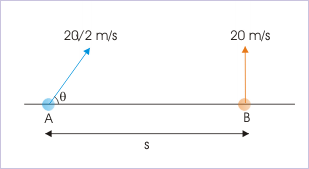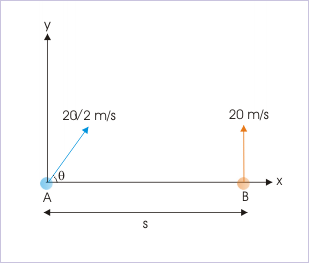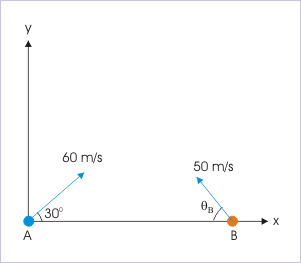# 5.9 Collision of projectiles  (Page 2/4)

 Page 2 / 4

${v}_{ABx}={v}_{Ax}-{v}_{Bx}=0$

## Relative motion in y-direction

If “ ${y}_{0}$ ” is the initial separation between projectiles, then for collision they should cover this separation with y-component of relative velocity. Since two projectiles are initiated at the same time instant, the time when collision occurs is given by :

$t=\frac{{y}_{0}}{{v}_{ABy}}$

where ${v}_{ABy}$ is the relative speed of approach of A with respect B. Note that time expression evaluates to same value whether we compute it with ${v}_{ABy}$ or ${v}_{BAy}$ . On the other hand, if there is no initial separation in y – direction, the projectiles should cover same vertical distance for all time intervals. It is so because projectiles have to reach same vertical i..e y-position at the point of collision in two dimensional space. This means that relative velocity of projectiles in y-direction is zero for the condition of collision. Mathematically,

${v}_{ABy}={v}_{Ay}-{v}_{By}=0$

## Collision of projectiles initiated without vertical separation

In this case, there is no initial vertical separation. Such is the case, when projectiles are projected from same horizontal level. Both projectiles should rise to same height for all time. Clearly, relative velocity in vertical i.e y-direction is zero :

${v}_{ABy}={v}_{Ay}-{v}_{By}=0$

On the other hand, time of collision is obtained by considering relative motion in x-direction :

$t=\frac{{x}_{0}}{{v}_{ABx}}$

There are different cases for projection from the same horizontal level. Some important cases are : (i) one projectile is projected at certain angle to the horizontal while the other projectile is projected vertically and (ii) Both projectiles are projected at certain angles to the horizontal. Here, we shall work out examples for each of these cases.

Problem : Two projectiles “A” and “B” are projected simultaneously as shown in the figure. If they collide after 0.5 s, then determine (i) angle of projection “θ” and (ii) the distance “s”.The projectiles collide after 0.5 s.

Solution : We see here that projectile “A” is approaching towards projectile “B” in horizontal direction. Their movement in two component directions should be synchronized so that they are at the same position at a particular given time. There is no separation in vertical direction at the start of motion. As such, relative velocity in y-direction should be zero for collision to occur.The projectiles collide after 0.5 s.

${v}_{ABy}={u}_{Ay}-{u}_{By}=0$

$⇒20\sqrt{2}\phantom{\rule{1em}{0ex}}\mathrm{sin}\theta =20$

$⇒\mathrm{sin}\theta =\frac{1}{\sqrt{2}}=\mathrm{sin}{45}^{0}$

$⇒\theta ={45}^{0}$

In the x-direction, the relative velocity is :

${v}_{ABx}={u}_{Ax}-{u}_{Bx}=20\sqrt{2}\mathrm{cos}{45}^{0}-0=20\phantom{\rule{1em}{0ex}}m/s$

The distance “s” covered with the relative velocity in 0.5 second is :

$s={v}_{ABx}Xt=20X0.5=10m$

Problem : Two projectiles “A” and “B” are thrown simultaneously in opposite directions as shown in the figure. If they happen to collide in the mid air, then find the time when collision takes place.Two projectiles are thrown towards each other.

Solution : There is no separation in vertical direction at the start of motion. As such, relative velocity in y-direction should be zero for collision to occur.

${v}_{ABy}={u}_{Ay}-{u}_{By}=0$

$⇒{u}_{A}\mathrm{sin}{\theta }_{A}={u}_{B}\mathrm{sin}{\theta }_{B}$

Putting values,

$⇒\mathrm{sin}{\theta }_{B}=\frac{60}{50}X\mathrm{sin}{30}^{0}$

$⇒\mathrm{sin}{\theta }_{B}=\frac{3}{5}$

The projectiles move towards each other with the relative velocity in horizontal direction. The relative velocity in x-direction is :

${v}_{ABx}={u}_{Ax}-{u}_{Bx}=60\mathrm{cos}{30}^{0}+50\mathrm{cos}{\theta }_{B}$

In order to find relative velocity in x-direction, we need to know “ $\mathrm{cos}{\theta }_{B}$ ”. Using trigonometric relation, we have :

$\mathrm{cos}{\theta }_{B}=\sqrt{\left(1-\mathrm{sin}{}^{2}{\theta }_{B}\right)}=\sqrt{\left\{1-{\left(\frac{3}{5}\right)}^{2}\right\}}=\frac{4}{5}$

Hence,

$⇒{v}_{ABx}=60X\frac{\sqrt{3}}{2}+50X\frac{4}{5}=30\sqrt{3}+40=91.98\approx 92m/s$

We should now understand that projectiles move towards each other with a relative velocity of 92 m/s. We can interpret this as if projectile “B” is stationary and projectile “A” moves towards it with a velocity 92 m/s, covering the initial separation between two particles for collision to take place. The time of collision, therefore, is :

$⇒t=92/92=1s$

#### Questions & Answers

What are the system of units
Jonah Reply
A stone propelled from a catapult with a speed of 50ms-1 attains a height of 100m. Calculate the time of flight, calculate the angle of projection, calculate the range attained
Samson Reply
58asagravitasnal firce
Amar
water boil at 100 and why
isaac Reply
what is upper limit of speed
Riya Reply
what temperature is 0 k
Riya
0k is the lower limit of the themordynamic scale which is equalt to -273 In celcius scale
Mustapha
How MKS system is the subset of SI system?
Clash Reply
which colour has the shortest wavelength in the white light spectrum
Mustapha Reply
how do we add
Jennifer Reply
if x=a-b, a=5.8cm b=3.22 cm find percentage error in x
Abhyanshu Reply
x=5.8-3.22 x=2.58
sajjad
what is the definition of resolution of forces
Atinuke Reply
what is energy?
James Reply
Ability of doing work is called energy energy neither be create nor destryoed but change in one form to an other form
Abdul
motion
Mustapha
highlights of atomic physics
Benjamin
can anyone tell who founded equations of motion !?
Ztechy Reply
n=a+b/T² find the linear express
Donsmart Reply
أوك
عباس
Quiklyyy
Sultan Reply
Moment of inertia of a bar in terms of perpendicular axis theorem
Sultan Reply
How should i know when to add/subtract the velocities and when to use the Pythagoras theorem?
Yara Reply

### Read also:

#### Get the best Physics for k-12 course in your pocket!

Source:  OpenStax, Physics for k-12. OpenStax CNX. Sep 07, 2009 Download for free at http://cnx.org/content/col10322/1.175
Google Play and the Google Play logo are trademarks of Google Inc.

Notification Switch

Would you like to follow the 'Physics for k-12' conversation and receive update notifications?ByByBy Anonymous User